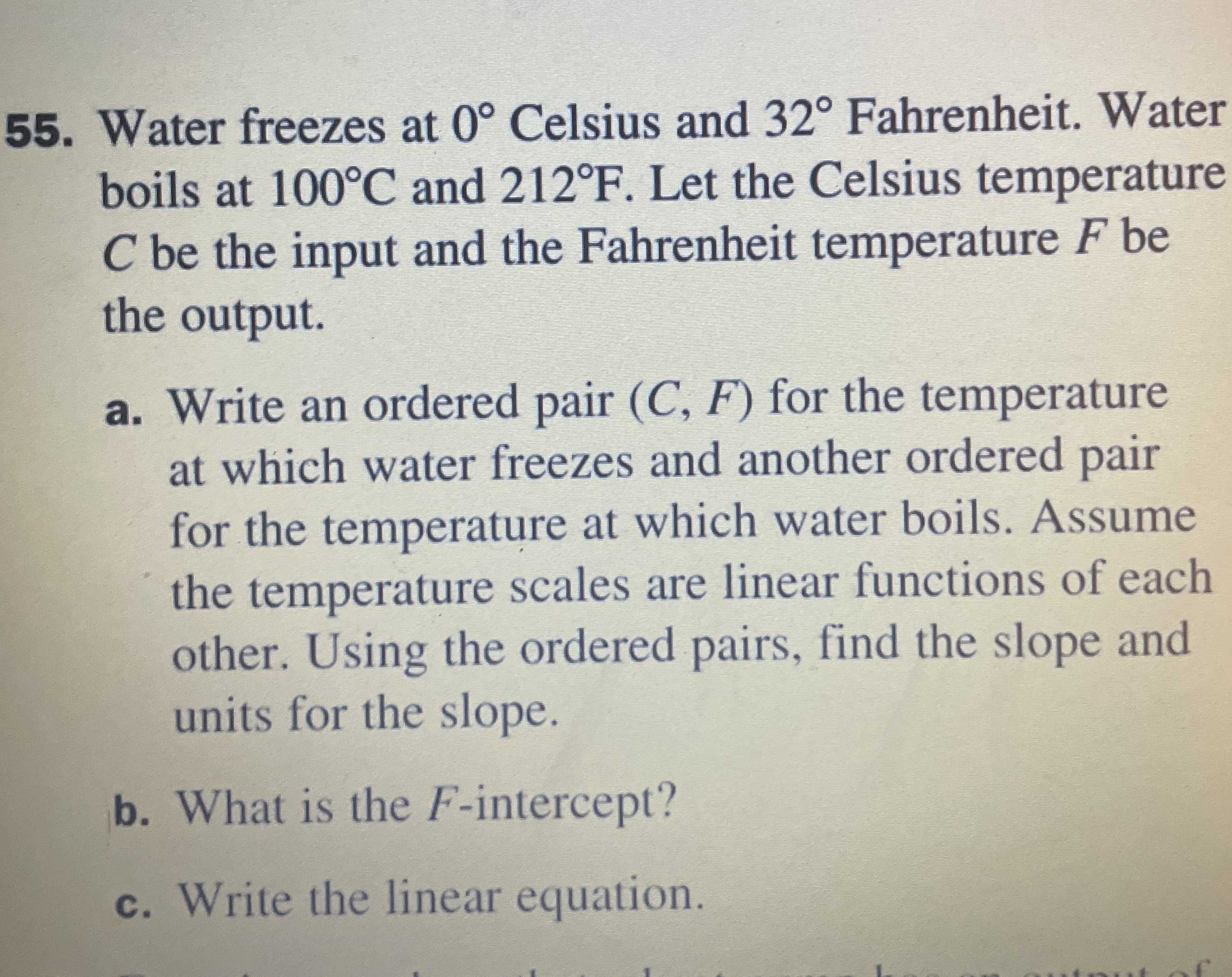### ¿Todavía tienes preguntas de matemáticas?

Pregunte a nuestros tutores expertos
Algebra
Pregunta55. Water freezes at $$0 ^ { \circ }$$ Celsius and $$32 ^ { \circ }$$ Fahrenheit. Water boils at $$100 ^ { \circ } C$$ and $$212 ^ { \circ } F$$ . Let the Celsius temperature $$C$$ be the input and the Fahrenheit temperature $$F$$ be the output.

a. Write an ordered pair $$( C , F )$$ for the temperature at which water freezes and another ordered pair for the temperature at which water boils. Assume the temperature scales are linear functions of each other. Using the ordered pairs, find the slope and units for the slope.

b. What is the $$F$$ -intercept?

c. Write the linear equation.

a)

ordered pair for the temperature at which water freezes = ( 0, 32 )

ordered pair for the temperature at which water boils = (100, 212 )

b.

F-intercept = 32

c.

Equation of the line:

$$y = 1.8 x + 32$$

or

$$y = \frac { 9 } { 5 } x + 32$$

Solución
View full explanation on CameraMath App.